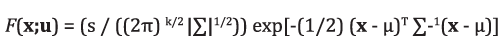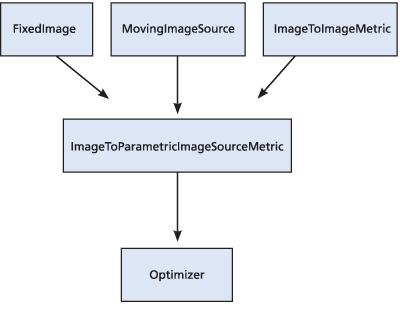# Adapting ITK Framework to Fit Parametric Image Models

January 15, 2011Fitting models of image pixel values to images is a task central to certain image analysis applications. For example, in fluorescence microscopy, light from an in-focus point source diffracts to an intensity pattern that approximately matches a 2D Gaussian function. Using this observation, methods have been developed for determining the positions of individual fluorescent molecules by fitting a 2D Gaussian function to the image . The mean (center) of the fitted Gaussian is taken to be the location of the molecule. Localization accuracy of as low as 1.5 nanometers has been reported using this method .

Despite having mature implementations of the necessary algorithmic foundations, the Insight Toolkit (ITK) does not currently have a mechanism to enable the fitting of image pixel value models to images. This document describes a set of classes that enable such fitting.

Parametric Models of Image Pixel Values
An image pixel value pattern can be modeled as a function F (x; u) where x is a spatial position and u is a set of function parameters that change the pattern. The general term “pixel value” is used because the classes proposed here impose no restriction on the types of data associated with pixels, e.g. scalar intensity values or vector values. The goal in fitting the function F (x;u) to an input image I (x) is to find the parameter vector u that minimizes a cost function defining how well the pixel values from the function match the pixel values of the input image. This is largely the same goal as in image registration. However, in registration, the parameter vector u is an argument to a spatial transformation T (x;u) such that the transformed input image I1(T (x;u)) closely matches another input image I2(x).

An example of a pixel value profile often used in image analysis is the Gaussian function. For the case of a normalized k-dimensional Gaussian function whose major axes are aligned with the Cartesian axes, F (x;u) has the formwhere s is a scaling factor, µ is the mean (center) vector, and is a diagonal matrix whose i-th diagonal element is the standard deviation σi of the Gaussian function along the i-th dimension. For this function, the parameter vector u is equal to {s, σi, …, σk, µ1,…, µk}. In ITK, the class itk::GaussianImageSource generates images whose intensities are defined by this function when its Normalized flag is set to 1.

Certain ITK image filters may also be considered parametric models of image pixel values. For these filters, the function is of the form F (x;u,I (x)) where u is a parameter vector as before and I(x) is the image input for the filter. In some applications it may be desirable to optimize the filter parameters u. For example, the itk::MultiplyByConstantImageFilter might be used to find an optimal intensity scaling factor for an image with respect to a particular objective function.

While ITK has parametric image sources, no unified interface for setting their parameters exists. This kind of interface, analogous to the interface currently found in subclasses of  itk::Transform, would enable application of the optimization routines in ITK to fitting image intensity models. We propose a new subclass of itk::ImageSource, called itk::ParametricImageSource, to provide a suitable interface. This class defines three methods:

 virtual void SetParameters(const ParametersType& p) {};virtual ParametersType GetParameters() const { return ParametersType(0); } virtual unsigned int GetNumberOfParameters() const { return 0; }

where the ParametersType is defined by

 typedef double        ParametersValueType; typedef Array ParametersType;

Subclasses with one or more parameters must override these three methods. For example, the itk::GaussianImageSource could be modified to be a subclass of itk::ParametricImageSource. In this case, the parameters for the Gaussian are the intensity scale, the mean, and the standard deviation. Subclasses of itk::ImageToImageFilter could also be modified to be a subclass of itk::ParametricImageSource with appropriate overriding of these three methods.

Connecting Parametric Image Sources to the Registration Framework
The image registration framework in ITK consists of several types of components: cost functions (e.g.,
itk::MeanSquareImageToImageMetric, itk::NormalizedCorrelationImageToImageMetric), spatial transforms (e.g., itk::AffineTransform, itk::BSplineDeformableTransform), interpolators (e.g., itk::LinearInterpolateImageFunction, itk::WindowsSincInterpolateImageFunction), and optimizers (e.g., itk::GradientDescentOptimizer, itk::PowellOptimizer). Briefly, an optimizer is a search routine that iteratively evaluates a cost function at different values of the transform parameters until the cost function is minimized (or possibly maximized). A cost function assigns a value to two images, a fixed image and a moving image, according to how well they match. The fixed image remains unmodified during registration while the moving image is warped according to the spatial transform. If the optimizer converges to a local minimum (or maximum), the transform and parameters found by the optimizer define a locally optimal warping from the moving image to the fixed image.

We would like to be able to reuse the existing subclasses of itk::ImageToImageMetric as cost functions in ITK to fit parametric image models to images. These cost functions have the methods

 MeasureType GetValue(const ParametersType &p) const; void GetDerivative(const ParametersType &p, DerivativeType &d) const; void GetValueAndDerivative(const ParametersType &p, MeasureType &v, DerivativeType &d) const;

that are called by optimizers. These methods pass their parameters argument to an itk::Transform object assigned to the image-to-image metric. This behavior makes it impossible to set parameters of an itk::ParametricImageSource through the existing registration framework. To overcome this limitation, we have defined a new subclass of itk::ImageToImageMetric, called itk::ImageToParametricImageSourceMetric, that lets us control where the parameters are passed.

Much like the existing itk::ImageToImageMetric subclasses, the itk::ImageToParametricImageSourceMetric is parameterized over fixed and moving image types. The moving image type, however, is expected to be a subclass of itk::ParametricImageSource, so it is actually an image source rather than static image data. The meaning of “moving” in this context means that the image intensities are changing values, rather than that the moving image is undergoing a spatial deformation.Figure 1: The itk::ImageToParametricImageSourceMetric adapts itk::ImageToImageMetrics for use in fitting image pixel value models. It sits between an existing itk::ImageToImageMetric and an itk::Optimizer.

The itk::ImageToParametricImageSourceMetric adapts a delegate itk::ImageToImageMetric for the purposes of computing the image match value. Parameters passed to the GetValue() method from the optimizer are forwarded to the itk::ParametricImageSource, which is updated before the delegate computes the cost function value. Figure 1 shows how the itk::ImageToParametricImageSourceMetric integrates into the registration framework.

We assume that the parametric image source has parameters that can accommodate any necessary spatial transformations. Therefore, the itk::ImageToParametricImageSourceMetric forces the delegate metric to use an itk::IdentityTransform. We do not make any assumptions about the size, spacing, or origin of the fixed or moving images, so an interpolator for the fixed image must be set using the method SetInterpolator().

The interface for the itk::ParametricImageSource does not currently provide a mechanism to retrieve partial derivative images that can be used for computing the gradient of the itk::ImageToImageMetric in the method GetDerivative(). Instead, we estimate the derivative via forward differences.

Because it may be desirable to optimize over a subset of the parameters in an itk::ParametricImageSource, the itk::ImageToParametricImageSourceMetric class also holds a mask vector that indicates which parameters should be optimized. We call these enabled parameters. The method itk::GetNumberOfParameters() reports the number of enabled parameters. This method tells the itk::Optimizer the dimensionality of the parameter space that it will explore, so the itk::Optimizer will pass arrays containing values for only the enabled parameters to the GetValue() method of itk::ImageToParametricImageSourceMetric. However, the SetParameters() method in itk::ParametricImageSource expects an array containing values for all the parameters. The simple solution is to retrieve the current parameters from the itk::ParametricImageSource, set the active parameters passed in by the itk::Optimizer, and then pass this modified parameter array back to the itk::ParametricImageSource using that class’s SetParameters() method. The class itk::ImageToParametricImageSourceMetric has its own SetParameters() method that takes care of this step.

Writing Adapter Classes for Existing Image Sources
A pragmatic way to implement image model fitting is to write classes that adapt existing image source to the itk::ParametricImageSource interface. As an example, the implementations of the key methods in the adapter class for the itk::GaussianImageSource are given below.

 template void ParametricGaussianImageSource ::SetParameters(const ParametersType& parameters) { unsigned int i; const unsigned int dimensions = itkGetStaticConstMacro(OutputImageDimension); ArrayType sigma, mean; for (i=0; iSetSigma(sigma); this->SetMean(mean); this->SetScale(parameters[2*dimensions]); }template typename ParametricGaussianImageSource ::ParametersType ParametricGaussianImageSource ::GetParameters() const { ParametersType params(GetNumberOfParameters()); ArrayType sigma = this->GetSigma(); ArrayType mean  = this->GetMean(); unsigned int i; const unsigned int dimensions = itkGetStaticConstMacro(OutputImageDimension); for (i=0; iGetScale(); return params; } template unsigned int ParametricGaussianImageSource ::GetNumberOfParameters() const { // Standard deviation vector, mean vector, // and scale. return 2* itkGetStaticConstMacro(OutputImageDimension) + 1; }

Fitting a 2D Gaussian Pixel Intensity Model to an Image
Several nanometer-resolution fluorescence microscopy imaging methods rely on fitting a 2D Gaussian to the pattern of image intensities produced by a single fluorescent molecule. Here, we show how this can be accomplished using ITK’s registration framework and the adapter classes presented in this document.

First, we include the necessary software components from the registration framework:

 #include #include #include

Next, we include the existing itk::GaussianImageSource along with the image-to-image metric adapter and the adapter for the itk::GaussianImageSource.

 #include #include #include

We then define some useful typedefs:

 typedef double PixelType; typedef itk::Image GaussianImageType; typedef itk::GaussianImageSource GaussianImageSourceType; typedef itk ::ParametricGaussianImageSource ParametricGaussianImageSourceType; typedef ParametricGaussianImageSourceType ::ParametersType ParametersType; typedef itk ::LinearInterpolateImageFunction InterpolatorType; typedef itk ::ImageToParametricImageSourceMetric MetricType; typedef itk::MeanSquaresImageToImageMetric DelegateMetricType; typedef itk::AmoebaOptimizer AmoebaOptimizerType;

Assuming we have a 2D Image named input that has a 2D Gaussian intensity profile, we next want to set
up an itk::ParametricGaussianImageSource that will be used in the fitting.

 ParametricGaussianImageSourceType::Pointer fittingImageSource = ParametricGaussianImageSourceType::New(); fittingImageSource->SetSize(input->GetSize()); fittingImageSource->SetSpacing(input->GetSpacing()); fittingImageSource->SetOrigin(input->GetOrigin());

We set up the metric with an interpolator, delegate metric, fixed image, and moving image source.

 InterpolatorType::Pointer interpolator = InterpolatorType::New(); DelegateMetricType::Pointer delegate = DelegateMetricType::New(); MetricType::Pointer metric = MetricType::New(); metric->SetInterpolator(interpolator); metric->SetDelegateMetric(delegate); metric->SetFixedImage(originalImage->GetOutput()); metric->SetFixedImageRegion(originalImage-> GetOutput()->GetLargestPossibleRegion()); metric->SetMovingImageSource(fittingImage);

Finally, we set up the optimizer and start optimization.

 amoebaOptimizerType::Pointer amoebaOptimizer = AmoebaOptimizerType::New(); amoebaOptimizer->SetCostFunction(metric);amoebaOptimizer-> SetInitialPosition(startingParameters); amoebaOptimizer->StartOptimization();

A source file GaussianFitting2DTest.cxx that accompanies the Insight Journal version of this article (http://hdl.handle.net/10380/3167) contains a test of the classes for image model fitting presented here. It generates an image of a Gaussian intensity profile using the itk::GaussianImageSource with known mean, standard deviation, and scale, then fits an itk::ParametricGaussianImageSource to this image. The test shows that the known Gaussian parameters can be successfully recovered using both the itk::AmoebaOptimizer and itk::ConjugateGradientOptimizer.

References
   E. Betzig, G. H. Patterson, R. Sougrat, O. Wolf Lindwasser, S. Olenych, J. S. Bonifacino, M. W. Davidson, J.
Lippincott-Schwartz, and H. F. Hess. Imaging Intracellular Fluorescent Proteins at Nanometer Resolution. Science,
313(5793):1642–1645, 2006.
   R. E. Thompson, D. R. Larson, and W. W. Webb. Precise nanometer localization analysis for individual fluorescent
probes. Biophysical Journal, 82(5):2775–2783, 2002.
   A. Yildiz, J. N Forkey, S. A McKinney, T. Ha, Y. E Goldman, and P. R Selvin. Myosin v walks hand-over-hand:
single fluorophore imaging with 1.5-nm localization. Science, 300(5628):2061–2065, 2003.

Acknowledgements
This work was funded by NIH grant P41-EB002025-25A1.Cory Quammen is a software engineer and graduate student in the Department of Computer Science at the University of North Carolina at Chapel Hill. His work involves analysis of fluorescence microscopy images.Russell Taylor is a Research Professor of Computer Science, Physics & Astronomy, and Applied & Materials Sciences at the University of North Carolina at Chapel Hill.  He is the co-director of the UNC NIH National Research Resource for Computer Integrated Systems for Microscopy and Manipulation. His research interests include Scientific Visualization, Distributed Virtual Worlds, Haptic Display, and Interactive 3D Computer Graphics.

Tags: